# Regular Expression in Java

## Regular Expression in Java with Examples

In this article, I am going to discuss Regular Expression in Java with Examples. Please read our previous article where we discussed Internationalization in Java with Examples. At the end of this article, you will understand what is Regular Expression in Java and when and how to use Regular Expression in Java applications.

##### What is Regular Expression in Java?

A Regular Expression is an expression that represents a group of strings according to a particular pattern. Java Regex API provides interface and classes in java.util.regex package.

Example: We can write a Regular Expression to represent all valid mail ids. We can write a Regular Expression to represent all valid mobile numbers.

##### The main important application areas of Regular Expression are:
1. To implement validation logic.
2. To develop Pattern matching applications.
3. To develop translators like compilers, interpreters, etc.
4. To develop digital circuits.
5. To develop communication protocols like TCP/IP, UDP, etc.
##### Example to Understand Regular Expression in Java
```import java.util.regex.*;
public class RegExpression1
{
public static void main (String args[])
{
int count = 0;
Pattern p = Pattern.compile ("ab");
Matcher m = p.matcher ("abbbabbaba");
while (m.find ())
{
count++;
System.out.println (m.start () + "-----" + m.end () + "-----" + m.group ());
}
System.out.println (("the no of occurences : " + count));
}
}
```
###### Output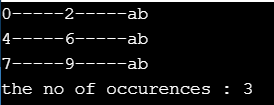##### Pattern Class in Java

A Pattern object represents a “compiled version of Regular Expression” in Java. We can create a Pattern object by using the compile() method of the Pattern class.

Syntax: public static Pattern compile(String regex);

Example: Pattern p = Pattern.compile(“ab”);

##### Methods of Pattern Class in Java
1. static Pattern compile(String regex): It is used to compile the given regex and returns the instance of the Pattern.
2. Matcher matcher(CharSequence input): It is used to create a matcher that matches the given input with the pattern.
3. Static Boolean matches(String regex, CharSequence input): It actually works as the combination and matches of compile and matcher methods. It is used to compile the regular expression and matches the given input with the pattern.
4. String[] split(CharSequence input): It splits the given input string around matches of the given pattern.
5. String pattern(): It is used to return the regex pattern.
##### Matcher Class in Java

A Matcher object can be used to match character sequences against a Regular Expression. We can create a Matcher object by using the matcher() method of the Pattern class.

Syntax: public Matcher matcher(String target);

Example: Matcher m = p.matcher(“abbbabbaba”);

##### Important methods of Matcher Class in Java
1. boolean find(): It attempts to find the next match and return true if it is available otherwise returns false.
2. int start(): Returns the start index of the match.
3. int end(): Returns the offset(equalize) after the last character matched (or) Returns the end index of the match.
4. String group(): Returns the matched Pattern.
##### Character Classes in Java

Character classes are constructs that enable you to specify a match against multiple characters instead of just one.
[abc] Either ‘a’ or ’b’ or ‘c’
[^abc] Except ‘a’ and ‘b’ and ‘c’
[a-z] Any lowercase alphabet symbol
[A-Z] Any uppercase alphabet symbol
[a-zA-z] Any alphabet symbol
[0-9] Any digit from 0 to 9
[a-zA-Z0-9] Any alphanumeric character

##### Example to Understand Character Classes in Java
```import java.util.regex.*;
public class RegExpression2
{
public static void main (String[]args)
{
System.out.println (Pattern.matches ("[amn]", "abcd"));
System.out.println (Pattern.matches ("[amn]", "a"));
System.out.println (Pattern.matches ("[amn]", "ammmna"));
}
}
```
###### Output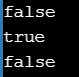##### Predefined Character Classes in Java

You also have a number of predefined character classes that provide you with a shorthand notation for commonly used sets of characters.
\s Space Character
\d Any digit from 0 to 9[0-9]
\w Any word character[a-zA-Z0-9]
. Any character including special character

##### Example to Understand Predefined Character Classes in Java
```import java.util.regex.*;
public class RegExpression3
{
public static void main (String[]args)
{
Pattern p = Pattern.compile ("\\D{3}\\d{3}");
Matcher m = p.matcher ("ABC123Xabc456UDEF789");
int i = 0;
while (m.find ())
{
i++;
System.out.println (i + "th subsequence : " + m.group ());
}
}
}
```
###### Output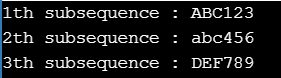##### Quantifiers in Java

Quantifiers can be used to specify the number of characters to match.
a Exactly one ‘a’
a+ At least one ‘a’
a* Any number of a’s including zero number
a? At most one ‘a’

##### Example to Understand Quantifiers in Java
```import java.util.regex.*;
public class RegExpression4
{
public static void main (String args[])
{
Pattern p = Pattern.compile ("a+");
Matcher m = p.matcher ("abaabaaab");
while (m.find ())
{
System.out.println (m.start () + "-----" + m.group ());
}
}
}
```
###### Output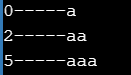##### Pattern class split() method in Java

Pattern class contains a split() method to split the given string against a regular expression.

##### Example to Understand Pattern class split() method in Java
```import java.util.regex.*;
public class RegExpression5
{
public static void main (String args[])
{
Pattern p = Pattern.compile ("\\s");
String[] s = p.split ("Hello World Welcome to Java Programming Language");
for (String s1:s)
{
System.out.println (s1);
}
}
}
```
###### Output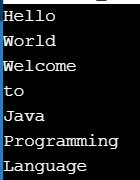##### String Class split() Method in Java

The string class also contains a split() method to split the given string against a regular expression.

##### Example to Understand String Class split() Method in Java
```import java.util.regex.*;
public class RegExpression6
{
public static void main (String args[])
{
String[] s1 = s.split ("\\.");
for (String s2:s1)
{
System.out.println (s2);
}
}
}
```
###### OutputNote: The String class split() method can take a regular expression as an argument whereas the pattern class split() method can take the target string as the argument.

##### String Tokenizer in Java

This class is present in the java.util.package. It is a specially designed class to perform string tokenization. The default regular expression for the String Tokenization is space.

##### Example to Understand String Tokenizer in Java
```import java.util.*;
public class RegExpression7
{
public static void main (String args[])
{
StringTokenizer st = new StringTokenizer ("Welcome to Java Programming Language");
while (st.hasMoreTokens ())
{
System.out.println (st.nextToken ());
}
}
}
```
###### Output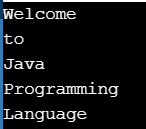##### Example to Understand String Tokenizer in Java
```import java.util.*;
public class RegExpression8
{
public static void main (String args[])
{
StringTokenizer st = new StringTokenizer ("1,99,988", ",");
while (st.hasMoreTokens ())
{
System.out.println (st.nextToken ());
}
}
}
```
###### Output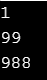##### Write a Regular Expression to represent all valid identifiers in Java

Rules: The allowed characters are :

1. a to z, A to Z, 0 to 9, -, #
2. The 1stcharacters should be alphabet symbols only.
3. The length of the identifier should be at least 2.
```import java.util.regex.*;
public class RegExpression9
{
public static void main (String[] args)
{
String name = "Abc1988";
Pattern p = Pattern.compile ("[a-zA-Z][a-zA-Z0-9-#]+");
// (or) Pattern p = Pattern.compile("[a-zA-Z][a-zA-Z0-9-#][a-zA-Z0-9-#]*");
Matcher m = p.matcher (name);
if (m.find () && m.group ().contentEquals (name))
{
System.out.println ("Valid Identifier");
}
else
{
System.out.println ("Invalid Identifier");
}
}
}
```

Output: Valid Identifier

##### Write a Regular Expression to represent all mobile numbers in Java

Rules:

1. Should contain exactly 10 digits.
2. The 1st digit should be 7 to 9.
```import java.util.regex.*;
public class RegExpression10
{
public static void main (String[]args)
{
String MobileNumber = "6709123456";
//Pattern p = Pattern.compile("[7-9][0-9][0-9][0-9][0-9][0-9][0-9][0-9][0-9][0-9]");
Pattern p = Pattern.compile ("[7-9][0-9]{9}");
Matcher m = p.matcher (MobileNumber);
if (m.find () && m.group ().equals (MobileNumber))
{
System.out.println ("Valid Number");
}
else
{
System.out.println ("Invalid Number");
}
}
}
```

Output: Invalid Number

##### Write a regular expression to represent all Mail Ids in Java
```import java.util.regex.*;
public class RegExpression11
{
public static void main (String[]args)
{
String EmailId = "info@dotnettutorials.net";
Pattern p = Pattern.compile ("[a-zA-Z][a-zA-Z0-9-.]*@[a-zA-Z0-9]+([.][a-zA-z]+)+");
Matcher m = p.matcher (EmailId);
if (m.find () && m.group ().contentEquals (EmailId))
{
System.out.println ("Valid Mail ID");
}
else
{
System.out.println ("Invalid Mail ID");
}
}
}
```

Output: Valid Mail ID

##### Write a program to extract all valid mobile numbers from a file in Java
```import java.util.regex.*;
import java.io.*;
public class RegExpression12
{
public static void main (String[]args) throws IOException
{
PrintWriter out = new PrintWriter ("output.text");
Pattern p = Pattern.compile ("[7-9][0-9]{9}");
while (line != null)
{
Matcher m = p.matcher (line);
while (m.find ())
{
out.println (m.group ());
}
}
out.flush ();
}
}
```
###### Output

input.txt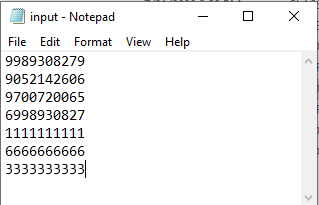Output.txt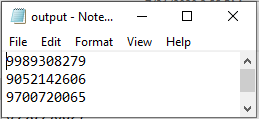##### Write a program to display all .txt file names present in G:\JAVA\Demo in Java
```import java.util.regex.*;
import java.io.*;
public class RegExpression13
{
public static void main (String[]args) throws IOException
{
int count = 0;
Pattern p = Pattern.compile ("[a-zA-Z0-9-\$.]+[.]txt");
File f = new File ("G:\\JAVA\\Demo");
String[] s = f.list ();
for (String s1:s)
{
Matcher m = p.matcher (s1);
if (m.find () && m.group ().equals (s1))
{
count++;
System.out.println (s1);
}
}
System.out.println ("No. of Files : " + count);
}
}
```
###### Output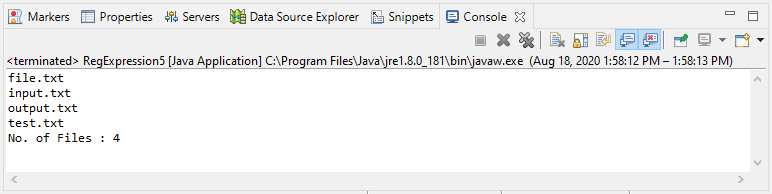In the next article, I am going to discuss Parallel Programming in Java with Examples. Here, in this article, I try to explain Regular Expression in Java with Examples. I hope you enjoy this Regular Expression in Java with Examples article.